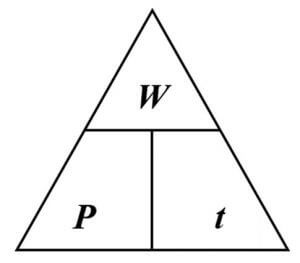# Power with Work Calculator

Use power calculator physics to find p=w/t by filling the following input box.

Formula Work = Power * Time## Formula

The formula of power, work, and time can be derived from each other as:

Power:
P = W/T

Work:
W = P*T

Time:
T = W/P

Where,
P = Power,
W = Work,
T = Time.

Time and work calculator uses all of the above formulas to calculate power, time, and work.

The power work time calculator simplifies the relationship between power, work, and time by providing the calculations of all three quantities in a single space. This work and power calculator finds the value of

We will explain power definition, how to find time with power and work without using physics power calculator, and how to use power formula physics calculator.

## What is power?

Power is the time rate at which work is done or energy is transferred. In short, power is the derivative of work with respect to time. The SI unit of power is Watt W or Joule per second J/s.

The following picture shows the relationship between work, power and time.## How to find power with work and time?

Finding power without using an online power with work calculator is not that difficult. To calculate time, power, or work, follow the steps below.

Example:
Calculate the power required to do a work of 25 J in 30 seconds of time.

Solution:

Step 1: Identify and write down the values.

Work (W) = 25 J
Time (T) = 30 s

Step 2: Apply Formula

Power = Work/Time (P = W/T)
P = 25/30
P = 0.83 W
Power (P) = 0.83 Watts

Verify the result using work power and energy calculator above.

1. How to Calculate Power Based on Work and Time - dummies.com
2. Calculate Power from Work and Time. flexbooks.ck12.org